Bitmap functions library Jiri Klimes xklime00@stud.fee.vutbr.cz Department of Computer Science & Engineering Technical University of BrnoBrno, Czech Republic1. Introduction

The goal of this contribution is to present the overview of basic algorithms for image processing. For the digital processing purposes, the image is stored as a digital raster containing picture elements. From the mathematical view point the image could be defined as continuous function of two variables – image function f(x, y). In computer graphics, RGB images with 24 bits per image element are normally used. Black and white image format (grayscale) with 8 bits per image element are also used, especially in technical applications.

Image processing is discipline of technology focused in processing raster images (bitmaps) on a computer. Several types of algorithms are used to achieve required changes in images. For example geometrical transformations to change the shape of images. For image processing, images are treated as 2-dimensional signal, so theory of signal processing is often used. This approach is used in image filtering and processing of spatial spectra. Significant importance in image processing have frequency transforms like Fourier transform. Image 1 shows a general approach to image processing.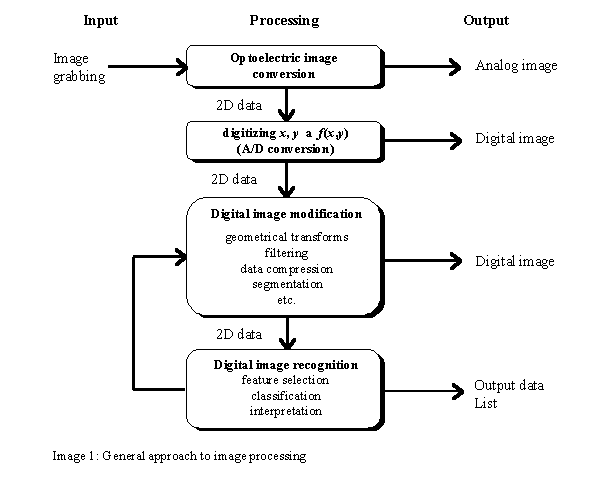Image processing that results in human-perceptible images would not be possible to perform without considering features of human visual system. At a first glance this assumption is very simple and natural. However, it has important implications. Let us ask the following questions:

• What differences in intensity is the human visual system able to distinguish?
• What is the spatial resolution of the human visual system?
• How precisely is the visual system able to measure distances in the image?
• What is the role of human colour vision?

For example, figure 2 shows a grey rectangle that appears to be lighter on a dark background and darker on a light background. This phenomenon disappears only if the two rectangles merge. The human visual system is able to distinguish about 2% difference in lightness in broad range of image intensities. Many other features of human visual system exist, that are generally necessary to consider, however, in some cases imperfections in visual systems can even make image processing easier.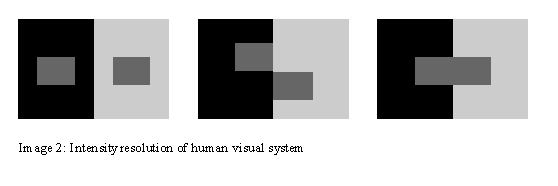1. Image Processing Algorithms

Image processing deals with offers several different image processing algorithms. The algorithms could be subdivided in the following groups based on their approach to image elements (picture elements, pixels):

1. Point Algorithms – Changes pixels values based on their original values or their position in the image.
2. Area Algorithms – Change pixels values based on their original values and pixels in their neighbourhood.
3. Frame Algorithms – The values of pixels in the images is based on the values of pixels from several source images.
4. Geometrical Algorithms – These algorithms change geometrical properties (pixel placement) in the images.
5. Miscellaneous – Various algorithms that by their nature do not fall in any of the algorithms from the previous groups.

1. Point Algorithms

Each ”point” in the image is represented by small element – pixel. Point algorithms calculate values on the pixels in the resulting image based on the values in the original image and on the position in the original image. General point algorithm representation could be expressed by the following equation: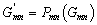. (1)

Indexes m, n of the function P express the explicit dependency of the results on the pixel position. If the function is not depending on the position, the function could be marked as homogenous and it could be expressed in the following way: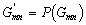. (2)

The typical point operations are:

• Adjustment of the lightness in the image
• Adjustment of the contrast in the image
• Histogram equalisation
• Creation of a negative image

1. Area Algorithms

Area algorithms calculate pixel values based on the group of pixels in the original image. The group could be defined (without any loss of generality) as 2-dimensional array with the same odd dimensions in both directions. The corresponding pixel in the resulting image is located in the centre of such array. The array is usually relatively small. In practise, dimensions like 3x3, 5x5, or 7x7 are used. Only exceptionally larger areas are used. The are algorithms are more complex and more powerful than point algorithms and allow for solving the following tasks:

• Noise filtering
• Correction of singular imperfections caused by the grabbing process, image transfer, or digital storage.
• Compensations of wrongly focused, or otherwise corrupt images – this type of operations is generally called image restorations as it attempts to restore the original images from degraded images.
• Enhancement or suppression of image details.
• Detection of simple local features in the images, such as edges, corners, or e.g. blobs with similar intensity.

Area algorithms usually perform tasks that are generally called image filtering.

2.3 Frame Algorithms

Frame algorithms are algorithms that work with more images at the same time. The purpose is to combine more images at the same time. The algorithms always concern two or more pixels located at the corresponding positions in the source images. Most often the operations are binary (concern two source images). This type of algorithms could be expressed as: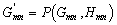. (3)

Algorithms from this group could be used for:

• Image subtraction
• Image multiplication
• a -blending (linear combination of image pixels)
• Bitwise logical image operations (OR, AND, XOR)

1. Geometrical Algorithms

Geometrical algorithms generally change the shape of the images. These changes are done through changing of number of pixels, location of pixels, or mutual position of pixels base or some geometrical transform. Often used are the following Geometrical transforms:

• Correction of geometrical defects in the images
• Image rotation
• Image mirroring
• As pre-processing step of other algorithms
• Preparing images for printing

1. Implementation of Selected Algorithms
2.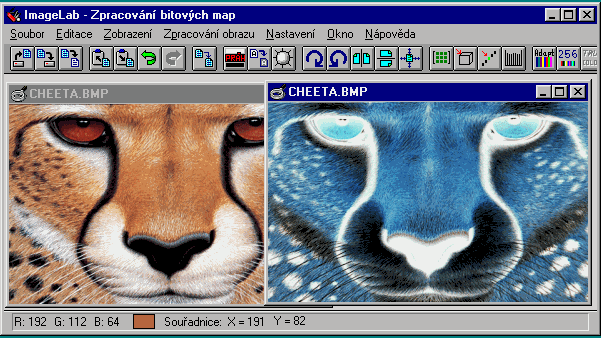Image 3: Negative of imageImage 4: Edge detection with Laplace operator using convolution

The two pictures above show the screen of the program with implemented algorithms. This program I have implemented in C++ for Windows. The image processing algorithms form a DLL (dynamic-link library), which has been implemented in ANSI C for better portability.

On image 3, there is example of the point algorithm – negative of image. Image 4 is for documentation of area algorithms. It shows usage of convolution of the image with convolution mask. The mask, in this case, represents Laplace’s operator and it’s size is 5 ´  5. This operation is used for finding edges in images. It is direction invariant, i.e., it detects edges of all directions.

3. Conclusions
4. This article tried to present a brief explanation of image processing algorithms. As for implemented library of algorithms, the main contribution is independence of algorithms. The implemented library represents only the computation part of algorithms. All dependent parts, such as manipulating with different graphic formats, allocation of memory etc., are implemented in application program separately from the algorithms. So the library can be modified to work on digital signal processors with small effort.

5. Literature

[Jä hne93] Jä hne, B.: Digital Image Processing: Concepts, Algorithms, and Scientific Applications, 2, Springer-Verlag, Berlin, 1993.

[Levine85] Levine, M. D.: Vision in Man and Machine, McGraw-Hill, New York, 1985.

[Ružic95] Ružický, E., Ferko, A.: Počítačová grafika a spracovanie obrazu, SAPIENTIA, Bratislava, 1995.

[Young86] Young, T. Y., Fu, K.: Handbook of Pattern Recognition and Image Processing, Academic Press, San Diego, 1986.

[Žára92] Žára, J. a kol.: Počítačová grafika – principy a algoritmy, Grada, 1992.